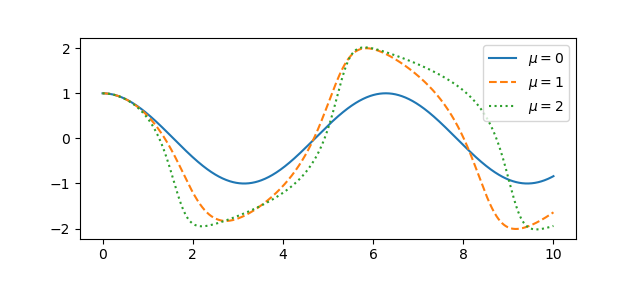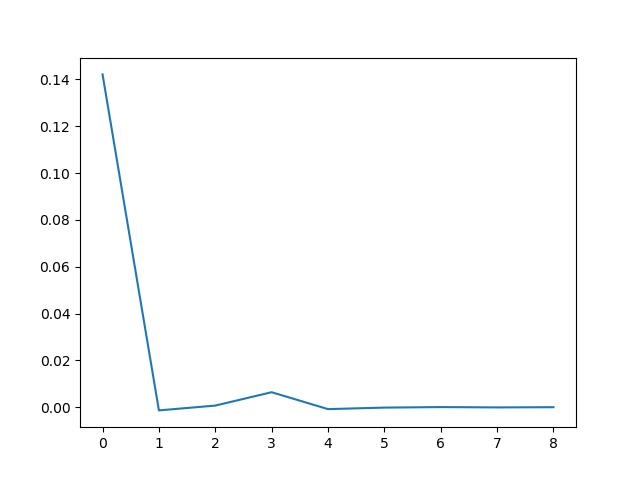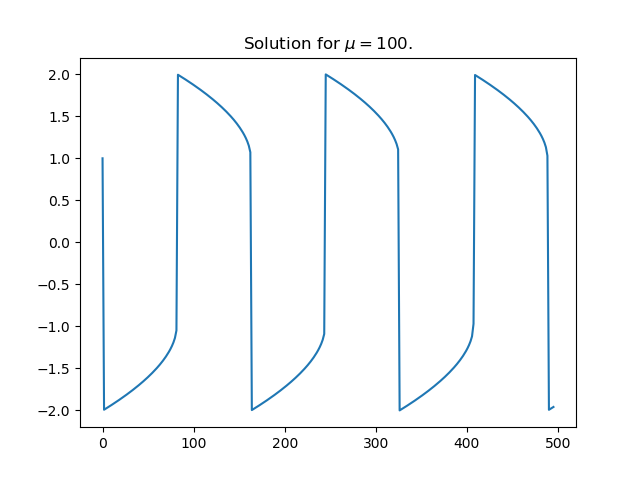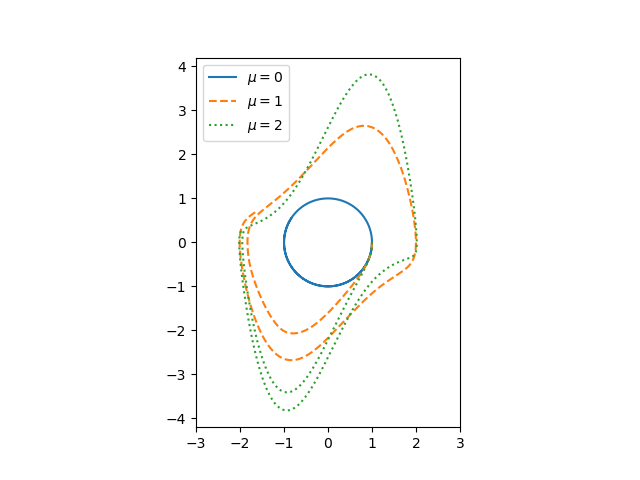# More efficient way to sum a list comprehension

List comprehensions in Python let you create a list declaratively, much like the way you would describe the set in English. For example,

    [x**2 for x in range(10)]

creates a list of the squares of the numbers 0 through 9.

If you wanted the sum of these numbers, you could of course say

    sum( [x**2 for x in range(10)] )

but in this case the brackets are unnecessary. The code is easier to read and more efficient if you omit the brackets.

    sum( x**2 for x in range(10) )

With the brackets, Python constructs the list first then sums the elements. Without the brackets, you have a generator expression. Python will sum the values as they’re generated, not saving all the values in memory. This makes no difference for a short list as above, but with a large list comprehension the generator expression could be more efficient.

# Area of sinc and jinc function lobes

Someone left a comment this morning on my blog post on sinc and jinc integrals regarding the area of the lobes.

It would be nice to have the values of integrals of each lobe, i.e. integrals between 0 and multiples of pi. Anyone knows of such a table?

This post will include Python code to address that question. (Update: added asymptotic approximation. See below.)

First, let me back up and explain the context. The sinc function is defined as 

sinc(x) = sin(x) / x

and the jinc function is defined analogously as

jinc(x) = J1(x) / x,

substituting the Bessel function J1 for the sine function. You could think of Bessel functions as analogs of sines and cosines. Bessel functions often come up when vibrations are described in polar coordinates, just as sines and cosines come up when using rectangular coordinates.

Here’s a plot of the sinc and jinc functions:The lobes are the regions between crossings of the x-axis. For the sinc function, the lobe in the middle runs from -π to π, and for n > 0 the nth lobe runs from nπ to (n+1)π. The zeros of Bessel functions are not uniformly spaced like the zeros of the sine function, but they come up in application frequently and so it’s easy to find software to compute their locations.

First of all we’ll need some imports.

    from scipy import sin, pi
from scipy.special import jn, jn_zeros


The sinc and jinc functions are continuous at zero, but the computer doesn’t know that . To prevent division by zero, we return the limiting value of each function for very small arguments.

    def sinc(x):
return 1 if abs(x) < 1e-8 else sin(x)/x

def jinc(x):
return 0.5 if abs(x) < 1e-8 else jn(1,x)/x


You can show via Taylor series that these functions are exact to the limits of floating point precision for |x| < 10-8.

Here’s code to compute the area of the sinc lobes.

    def sinc_lobe_area(n):
n = abs(n)
integral, info = quad(sinc, n*pi, (n+1)*pi)
return 2*integral if n == 0 else integral


The corresponding code for the jinc function is a little more complicated because we need to compute the zeros for the Bessel function J1. Our solution is a little clunky because we have an upper bound N on the lobe number. Ideally we’d work out an asymptotic value for the lobe area and compute zeros up to the point where the asymptotic approximation became sufficiently accurate, and switch over to the asymptotic formula for sufficiently large n.

    def jinc_lobe_area(n):
n = abs(n)
assert(n < N)
integral, info = quad(jinc, jzeros[n-1], jzeros[n])
return 2*integral if n == 0 else integral


Note that the 0th element of the array returned by jn_zeros is the first positive zero of J1; it doesn’t include the zero at the origin.

For both sinc and jinc, the even numbered lobes have positive area and the odd numbered lobes have negative area. Here’s a plot of the absolute values of the lobe areas.## Asymptotic results

We can approximate the area of the nth lobe of the sinc function by using a midpoint approximation for 1/x. It works out that the area is asymptotically equal toWe can do a similar calculation for the area of the nth jinc lobe, starting with the asymptotic approximation for jinc given here. We find that the area of the nth lobe of the jinc function is asymptotically equal toTo get an idea of the accuracy of the asymptotic approximations, here are the results for n=100.

    sinc area:      0.00633455
asymptotic:     0.00633452
absolute error: 2.97e-8
relative error: 4.69e-6

jinc area:      0.000283391
asymptotic:     0.000283385
absolute error: 5.66e-9
relative error: 2.00e-5


## More signal processing posts

 Some authors define sinc(x) as sin(πx)/πx. Both definitions are common.

 Scipy has a sinc function in scipy.special, defined as sin(πx)/πx, but it doesn’t have a jinc function.

# Minimizing context switching between shell and Python

Sometimes you’re in the flow using the command line and you’d like to briefly switch over to Python without too much interruption. Or it could be the other way around: you’re in the Python REPL and need to issue a quick shell command.

One solution would be to run your shell and Python session in different terminals, but let’s assume that for whatever reason you’re working in just one terminal. For example, maybe you want the output of a shell command to be visually close when you run Python, or vice versa.

## Calling Python from shell

You can run a Python one-liner from the shell by calling Python with the -c option. For example,

    $python -c "print(3*7)" 21  I hardly ever do this because I want to run more than a one-liner. What I find more useful is to launch Python with the -q option to suppress all the start-up verbiage and simply bring up a prompt. $ python -q
>>>>


More on this in my post on quiet modes.

## Calling shell from Python

If you run Python with the ipython command rather than the default python you get much better shell integration. IPython let’s you type a shell command at any point simply by preceding it with a !. For example, the following command tells us this is the 364th day of the year.

    In : ! date +%j
364


You can run some of the most common shell commands, such as cd and ls without even a bang prefix. These are “magic” commands that do what you’d expect if you forgot for a moment that you’re in a Python REPL rather than a command shell.

    In : cd ..
Out: '/mnt/c/Users'


IPython also supports other forms of shell integration such as capturing the output of a shell command as a Python variable, or using a Python variable as an argument to a shell command.

# Calculating the period of Van der Pol oscillators

A few days ago I wrote about how to solve differential equations with SciPy’s ivp_solve function using Van der Pol’s equation as the example. Van der Pol’s equation isThe parameter μ controls the amount of nonlinear damping. For any initial condition, the solution approach a periodic solution. The limiting periodic function does not depend on the initial condition  but does depend on μ. Here are the plots for μ  = 0, 1, and 2 from the previous post.A couple questions come to mind. First, how quickly do the solutions become periodic? Second, how does the period depend on μ? To address these questions, we’ll use an optional argument to ivp_solve we didn’t need in the earlier post.

## Using events in ivp_solve

For ivp_solve an event is a function of the time t and the solution y whose roots the solver will report. To determine the period, we’ll look at where the solution is zero; our event function is trivial since we want to find the roots of the solution itself.

Recall from the earlier post that we cast our second order ODE as a pair of first order ODEs, and so our solution is a vector, the function x and its derivative. So to find roots of the solution, we look at what the solver sees as the first component of the solver. So here’s our event function:

    def root(t, y): return y

Let’s set μ = 2 and find the zeros of the solution over the interval [0, 40], starting from the initial condition x(0) = 1, x‘(0) = 0.

    mu = 2
sol = solve_ivp(vdp, [0, 40], [1, 0], events=root)
zeros = sol.t_events


Here we reuse the vdp function from the previous post about the Van der Pol oscillator.

To estimate the period of the limit cycle we look at the spacing between zeros, and how that spacing is changing.

    spacing = zeros[1:] - zeros[:-1]
deltas = spacing[1:] - spacing[:-1]


If we plot the deltas we see that the zero spacings quickly approach a constant value. Zero crossings are half periods, so the period of the limit cycle is twice the limiting spacing between zeros.## Theoretical results

If μ = 0 the Van der Pol oscillator reduces to a simple harmonic oscillator and the period is 2π. As μ increases, the period increases.

For relatively small μ we can calculate the period as above, but as μ increases this becomes more difficult numerically . But one can easily show that the period is asymptotically

T ~ (3 – 2 log 2) μ

as μ goes to infinity. A more refined estimate due to Mary Cartwright is

T ~ (3 – 2 log 2) μ + 2π/μ1/3

for large μ.

## More VdP posts

 There is a trivial solution, x = 0, corresponding to the initial conditions x(0) = x‘(0) = 0. Otherwise, every set of initial conditions leads to a solution that converges to the periodic attractor.

 To see why large values of μ are a problem numerically, here’s a plot of a solution for μ = 100.The solution is differentiable everywhere, but the derivative changes so abruptly at the maxima and minima that it is discontinuous for practical purposes.

# Solving Van der Pol equation with ivp_solve

Van der Pol’s differential equation isThe equation describes a system with nonlinear damping, the degree of nonlinearity given by μ. If μ = 0 the system is linear and undamped, but as μ increases the strength of the nonlinearity increases. We will plot the phase portrait for the solution to Van der Pol’s equation in Python using SciPy’s new ODE solver ivp_solve.

The function ivp_solve does not solve second-order systems of equations directly. It solves systems of first-order equations, but a second-order differential equation can be recast as a pair of first-order equations by introducing the first derivative as a new variable.Since y is the derivative of x, the phase portrait is just the plot of (x, y).If μ = 0, we have a simple harmonic oscillator and the phase portrait is simply a circle. For larger values of μ the solutions enter limiting cycles, but the cycles are more complicated than just circles. These limiting cycles are periodic attractors: every non-trivial solution converges to the limit cycle.

Here’s the Python code that made the plot.

from scipy import linspace
from scipy.integrate import solve_ivp
import matplotlib.pyplot as plt

def vdp(t, z):
x, y = z
return [y, mu*(1 - x**2)*y - x]

a, b = 0, 10

mus = [0, 1, 2]
styles = ["-", "--", ":"]
t = linspace(a, b, 500)

for mu, style in zip(mus, styles):
sol = solve_ivp(vdp, [a, b], [1, 0], t_eval=t)
plt.plot(sol.y, sol.y, style)

# make a little extra horizontal room for legend
plt.xlim([-3,3])
plt.legend([f"$\mu={m}$" for m in mus])
plt.axes().set_aspect(1)


To plot the solutions as a function of time, rather than plotting phase portraits, change the line

    plt.plot(sol.y, sol.y, style)

to

    plt.plot(sol.t, sol.y, style)

and comment out the line setting xlim This gives the following plot.# Data Science and Star Science

I recently got a review copy of Statistics, Data Mining, and Machine Learning in Astronomy. I’m sure the book is especially useful to astronomers, but those of us who are not astronomers could use it as a survey of data analysis techniques, especially using Python tools, where all the examples happen to come from astronomy. It covers a lot of ground and is pleasant to read.# Generating Python code from SymPy

Yesterday I wrote about Householder’s higher-order generalizations of Newton’s root finding method. For n at least 2, defineand iterate Hn to find a root of f(x). When n = 2, this is Newton’s method. In yesterday’s post I used Mathematica to find expressions for H3 and H4, then used Mathematica’s FortranForm[] function to export Python code. (Mathematica doesn’t have a function to export Python code per se, but the Fortran syntax was identical in this case.)

Aaron Muerer pointed out that it would have been easier to generate the Python code in Python using SymPy to do the calculus and labdify() to generate the code. I hadn’t heard of lambdify before, so I tried out his suggestion. The resulting code is nice and compact.

    from sympy import diff, symbols, lambdify

def f(x, a, b):
return x**5 + a*x + b

def H(x, a, b, n):
x_, a_, b_ = x, a, b
x, a, b = symbols('x a b')
expr = diff(1/f(x,a,b), x, n-2) / \
diff(1/f(x,a,b), x, n-1)
g = lambdify([x,a,b], expr)
return x_ + (n-1)*g(x_, a_, b_)


This implements all the Hn at once. The previous post implemented three of the Hn separately.

The first couple lines of H require a little explanation. I wanted to use the same names for the numbers that the function H takes and the symbols that SymPy operated on, so I saved the numbers to local variables.

This code is fine for a demo, but in production you’d want to generate the function g once (for each n) and save the result rather than generating it on every call to H.

# Calling Python from Mathematica

The Mathematica function ExternalEvalute lets you call Python from Mathematica. However, there are a few wrinkles.

I first pasted in an example from the Mathematica documentation and it failed.

    ExternalEvaluate[
"Python",
{"def f(x): return x**2", "f(3)"}
]


It turns out you (may) have to tell Mathematica where to find Python. I ran the following, tried again, and the example worked.

    RegisterExternalEvaluator[
"Python",
"C:\\bin\\Anaconda3\\python.EXE"
]


You can also run Python with NumPy loaded using

    ExternalEvaluate["Python-NumPy", … ]


except that didn’t work the first time either. You have to register a Python-NumPy evaluator separately. So I ran

    RegisterExternalEvaluator[
"Python-NumPy",
"C:\\bin\\Anaconda3\\python.EXE"
]


and then tried again calling Python code using NumPy. But then there’s the question of how it imports NumPy. Does it simply run import numpy, or maybe from numpy import *, or maybe import numpy as np? It turns out the first possibility is what happens. So to print pi from NumPy, your code string needs to be numpy.pi.

You don’t need to use Python-NumPy if you just do your own importing. For example, this code returns π².

    ExternalEvaluate[
"Python",
"import numpy; x = numpy.pi; x**2"
]


And you can import any library you’d like, not just NumPy.

    ExternalEvaluate[
"Python",
"from sympy import isprime; isprime(7)"
]


Everything above applies to Mathematica 11.3 and Mathematica 12.

# Squircle perimeter and the isoparametric problem

If you have a fixed length of rope and you want to enclose the most area inside the rope, make it into a circle. This is the solution to the so-called isoparametric problem.

Dido’s problem is similar. If one side of your bounded area is given by a straight line, make your rope into a semi-circle.

This post looks at a similar problem for a squircle. Peter Panholzer mentioned the problem of finding the squircle exponent that led to the largest area in proportion to its arclength. This sounds like the isoparametric problem, but it’s not.

The isoparametric problem holds perimeter constant and lets the shape enclosed vary, maximizing the area. The question here is to hold the axes constant and maximize the ratio of the area to the perimeter. Panholzer reports the maximum occurs at p = 4.39365.

## Computing the perimeter

The volume of a squircle can be found in closed form, and I’ve mentioned the equation a few times, for example here. The perimeter, however, cannot be found in closed form, as far as I know, except for special exponents.

We can solve for y as a function of x and find the arclength using the formula taught in calculus courses. However, the derivative of y has a singularity at x = 1. By switching to polar coordinates, we can find arclength in terms of an integrand with no singularities. We can also simplify things a little by computing the total arclength as 4 times the arclength in the first quadrant; this avoids having to take absolute values.

The following Python code computes the perimeter and the ratio of the area to the perimeter.

    from scipy import sin, cos, pi
from scipy.special import gamma

def r(t, p):
return (cos(t)**p + sin(t)**p)**-(1/p)

def drdt(t, p):
deriv = (cos(t)**p + sin(t)**p)**(-1-1/p)
deriv *= cos(t)**(p-1)*sin(t) - sin(t)**(p-1)*cos(t)
return deriv

def integrand(t, p):
return (drdt(t,p)**2 + r(t,p)**2)**0.5

def arclength(p):
integral = quad(lambda t: integrand(t,p), 0, pi/2)
return 4*integral

def area(p):
return 4*gamma(1 + 1/p)**2 / gamma(1 + 2/p)

def ratio(p):
return area(p) / arclength(p)


Basic geometry tells us the ratio is 1/2 when p = 2 and we have a circle. The ratio is also 1/2 when p = ∞, i.e. when we have a square. We can use the code above to find that the ratio when p = 0.528627, so there is at least one local maximum for values of p between 2 and ∞.

Here’s a plot of the ratio of area to perimeter as a function of p.The plot is quite flat for large values of p, but if we zoom in we can see that there is a local maximum near 4.4.When I find the maximum of the ratio function using Brent’s method (scipy.optimize.brent) I find p = 4.39365679, which agrees with the value Panholzer stated.

Here’s a plot of the squircle corresponding to this value of p.## Back to the isoparametric problem

Now why doesn’t this contradict the isoparametric problem? Area scales quadratically but perimeter scales linearly. If you don’t hold perimeter constant, you can find a larger ratio of area to volume by making the perimeter larger. And that’s what we’ve done. When p = 2, we have a unit circle, with area π and perimeter 2π. When p = 4.39365679 we have area 3.750961567 and permimeter 7.09566295. If we were to take a circle with the same perimeter, it would have area 4.00660097, larger than the squircle we started with.

# Exploring the sum-product conjecture

Quanta Magazine posted an article yesterday about the sum-product problem of Paul Erdős and Endre Szemerédi. This problem starts with a finite set of real numbers A then considers the size of the sets A+A and A*A. That is, if we add every element of A to every other element of A, how many distinct sums are there? If we take products instead, how many distinct products are there?

## Proven results

Erdős and Szemerédi proved that there are constants c and ε > 0 such that

max{|A+A|, |A*A|} ≥ c|A|1+ε

In other words, either A+A or A*A is substantially bigger than A. Erdős and Szemerédi only proved that some positive ε exists, but they suspected ε could be chosen close to 1, i.e. that either |A+A| or |A*A| is bounded below by a fixed multiple of |A|² or nearly so. George Shakan later showed that one can take ε to be any value less than

1/3 + 5/5277 = 0.3342899…

but the conjecture remains that the upper limit on ε is 1.

## Python code

The following Python code will let you explore the sum-product conjecture empirically. It randomly selects sets of size N from the non-negative integers less than R, then computes the sum and product sets using set comprehensions.

    from numpy.random import choice

def trial(R, N):
# R = integer range, N = sample size
x = choice(R, N, replace=False)
s = {a+b for a in x for b in x}
p = {a*b for a in x for b in x}
return (len(s), len(p))


When I first tried this code I thought it had a bug. I called trial 10 times and got the same values for |A+A| and |A*A| every time. That was because I chose R large relative to N. In that case, it is likely that every sum and every product will be unique, aside from the redundancy from commutativity. That is, if R >> N, it is likely that |A+A| and |A*A| will both equal N(N+1)/2. Things get more interesting when N is closer to R.

## Probability vs combinatorics

The Erdős-Szemerédi problem is a problem in combinatorics, looking for deterministic lower bounds. But it seems natural to consider a probabilistic extension. Instead of asking about lower bounds on |A+A| and |A*A| you could ask for the distribution on |A+A| and |A*A| when the sets A are drawn from some probability distribution.

If the set A is drawn from a continuous distribution, then |A+A| and |A*A| both equal N(N+1)/2 with probability 1. Only careful choices, ones that would happen randomly with probability zero, could prevent the sums and products from being unique, modulo commutativity, as in the case R >> N above.

If the set A is an arithmetic sequence then |A+A| is small and |A*A| is large, and the opposite holds if A is a geometric sequence. So it might be interesting to look at the correlation of |A+A| and |A*A| when A comes from a discrete distribution, such as choosing N integers uniformly from [1, R] when N/R is not too small.